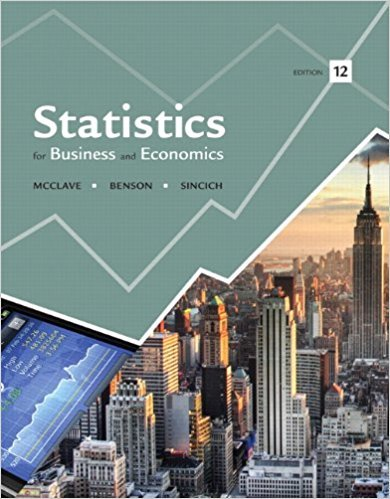×
Get Full Access to Statistics For Business And Economics - 12 Edition - Chapter 4 - Problem 79e
Get Full Access to Statistics For Business And Economics - 12 Edition - Chapter 4 - Problem 79e

×

# Flaws in plastic-coated wire. The British ColumbiaISBN: 9780321826237 51

## Solution for problem 79E Chapter 4

Statistics for Business and Economics | 12th Edition

• Textbook Solutions
• 2901 Step-by-step solutions solved by professors and subject experts
• Get 24/7 help from StudySoup virtual teaching assistantsStatistics for Business and Economics | 12th Edition

4 5 1 246 Reviews
16
5
Problem 79E

Problem 79E

Flaws in plastic-coated wire. The British Columbia Institute of Technology provides on its Web site (www.math.bcit.ca) practical applications of statistics at mechanical engineering firms. The following is a Poisson application. A roll of plastic-coated wire has an average of .8 flaws per 4-meter length of wire. Suppose a quality-control engineer will sample a 4-meter length of wire from a roll of wire 220 meters in length. If no flaws are found in the sample, the engineer will accept the entire roll of wire. What is the probability that the roll will be rejected? What assumption did you make to find this probability?

Step-by-Step Solution:

Step 1 of 1

The following is a Poisson application.

We have given a roll of plastic-coated wire has an average ofof wire.

Suppose an engineer will sample aof wire from a roll of wirein length.

If no flaws are found in the sample, the engineer will accept the entire roll of wire.

We are asked to find the probability that the roll will be rejected and state the assumption you have made to find the probability.

Letbe the number of flaws in aof wire.

Since we have given our process has a Poisson distribution and parameterisHence we can write,

A random variableis said to have a  Poisson probability distribution if and only if…………(1)

The roll will be rejected if there is at least one flaw in the sample of aof wire.

We need to findTherefore,Hence using equation (1), we can write,Hence the probability that the roll will be rejected isSince the process has a Poisson distribution, we have assumed that the flaws are randomly distributed and the 4-meter length of sample wire represent the entire roll.

Step 2 of 1

##### ISBN: 9780321826237

Unlock Textbook Solution